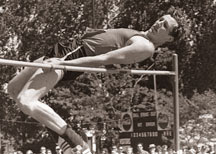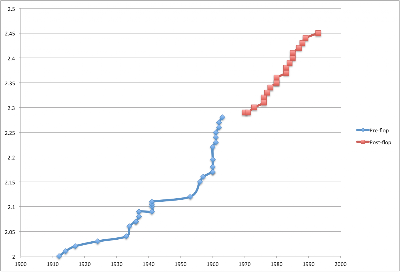Monday, August 1, 2011

Physics in Sports: The Fosbury FlopPhysics has greatly influenced the progress of most sports. There have been continual improvements in equipment for safety or performance as well as improvements in technique. I'd like to talk about some physics in sports over a series of posts. Here I'll talk about a technique improvement in High Jumping, the Fosbury Flop.

The Fosbury Flop came into the High Jumping scene in the 1968 Olympics, where Dick Fosbury used the technique to win the gold medal. The biggest difference between the Flop and previous methods is that the jumper goes over the bar upside down (facing the sky). This allows the jumper to bend their back so that their arms and legs drape below the bar which lowers the center of mass (See the picture above). Here is a video of the Fosbury Flop executed very well.Let’s assume Dick Fosbury is shaped like a semi-circle as he moves over the bar. The bar is indicated as a red circle, as this is a side view. From this diagram, we can guess his center of mass is probably near the marked 'x', since most of his mass is below the bar.

It is important to recall the definition of center of mass, which is the average location of all of the mass in an object.

$\vec{R} = \frac{1}{M} \int \vec{r} dm$
Note that this is a vector equation, and the integral should be over all of the mass elements. This integral gets easier because I'm going to assume that Dick Fosbury is a constant density semi-circle. This means that

$M = C*h$
where C is a constant equal to the ratio of the mass to the height, and

$dm = C * dh$.
This is a vector equation, so in principle we need to solve the x integral and the y integral; however, due to the symmetry about the y-axis, the x integral is zero. Finally we'll convert to polar coordinates, leaving us with:

$y = \frac{1}{C \pi R} \int_0^\pi R\sin{\theta} C R d\theta = \frac{1}{C \pi R} R (-\cos{\theta}) C R \bigg|_0^\pi = \frac{2R}{\pi}$
Ok, so this is the y-coordinate of the center of mass of our jumper relative to the bottom of the semi-circle. Now we need to calculate relative to the top of the bar, which is roughly the location of the top of the circle. We just need to subtract from R:

$R - \frac{2}{\pi} R = R * (1 - \frac{2}{\pi}) = \frac{h}{\pi} * (1 - \frac{2}{\pi})$
Now Dick Fosbury was 1.95m tall, which gives us a distance of 22.6 cm BELOW the bar! Of course he's not a semi-circle, but this isn't a terrible approximation, as you can see from the video linked above. Further, wikipedia mentions that some proficient jumpers can get their center of mass 20 cm below the bar, which matches pretty well with our guess.

A nifty technique in physics is looking at the point-particle system, which allows us to see the underlying motion of a system. If you’re not familiar with this method, you collect any given number of objects and replace them with a single point at the center of mass of the object. We can use energy conservation now for our point-mass instead of the entire body of the jumper.note

In this case, we can simply deal with the center of mass motion of the jumper. All of my kinetic energy will be converted to gravitational potential energy. Again this is an approximation because some energy is spent on forward motion, as well as the slight twisting motion which I'll ignore.

$E = \frac{1}{2} mv^2 = mgh$
Now let’s look at some data. Here is a plot of each world record in the high jump.The blue data show jumps before the Flop, and the red data show records after the Flop. **Note: In 1978, the straddle technique broke the world record, being the only non-flop technique to do so since 1968. Thanks Janne!** The Flop was revealed in 1968, so I’ll assume that all jumps before this year used a method where the center of mass of the jumper was roughly even with the bar, while all jumps after this year used the flop (see the previous note). Clearly something happened just before the Flop came out, and this is something called the Straddle technique.

I want to know the percent difference in the initial energies required, so I will calculate

$100\% * \frac{E_0-E_f}{E_0} = 100\% * \frac{mgh_0-mgh_f}{mgh_0} = 100\% * \frac{h_0-h_f}{h_0}$
where
$E_0$ is the initial energy without the force, err the flop, and
$E_f$ is the initial energy using the flop.

Since we are using the point-particle system, the gravitational potential energy only cares about the center of mass of the flopper, and we need to know the height of the center of mass for a 2.45m flop, which is the current world record.

This corresponds to a flop center of mass height of 2.25m, which gives us an 8.2% decrease in energy using the flop (versus a method where the center of mass is even with the bar)! The current world record is roughly 20 cm higher than it was when the flop came out. This could be due to athletes getting stronger, but this physics tells us that some of the height increase could have been from the technique change.

To sum up, the high jump competition, along with many other sports, is being exploited by physics!

[note] Here we're relying on the center of mass being equal to something called the center of gravity of the jumper. The center of mass is as defined above. The center of gravity is the average location of the gravitational force on the body. This happens to be the same as the center of mass if you assume we are in a uniform gravitational field, which is essentially true on the surface of the Earth.

1.The wikipedia page states the straddle technique took its last record 1978, at 2.34 (or 2.35 - it's slightly unclear writing), only 10cm lower than the current record. Which should mean either that flop jumpers aren't shifting their center of weight nearly as much as you estimate, or - more likely - straddle jumpers also manage some amount of weight shifting.

They also attribute the dominance of the flop to being easier to learn, rather than, or as much as being more effective. I can imagine that to be a rather important factor.

2.That's a good point Janne. I suppose I made it sound like all high jump records after the flop came out were floppers, which is incorrect. I'll add a note to the graph, thanks! I haven't looked into the straddle technique much, but from watching a couple of videos, I definitely agree that the straddle technique is trying to lower their center of mass. I was mainly trying to estimate by how much the center of mass of a jumper is lowered, and illustrate the idea that the center of mass of a high jumper actually goes below the bar.

I suppose a much more detailed analysis than this would be able to help in the case of flop v. straddle however. One thought I have is that although the flop may be easier to learn, good athletes will spend tireless hours learning any technique which will get them an edge. It seems to me that if the straddle method were slightly more efficient, more people would try using it. Since there's only been one record for the straddle since 1968, my guess is that at best it's about the same as the flop.

3.At the time I graduated from high school in the early 1960s, high-jumpers landed in a pit filled with sand softened with wood shavings mixed in. The toll on the body was a definite factor in meet strategy. At that time pole vaulters also landed in sand pits; imagine the beating their bodies took. It wasn't until the landing area was cushioned with nice thick foam that the Flop became feasible. Landing like that in a sand pit would likely eventually result in serious, permanent injury.

4.I like this concept. And this post.

5.It doesn't take a potential NASA employee to understand that the scissors technique was considerably less efficient than the straddle. In the scissors technique, the jumper went over the bar almost in a sitting position. when I started competing in grade school, I instinctively rejected that technique for a self-taught straddle. It seemed to me that scissors jumpers wasted energy by not only lifting their body mass higher, but also moving in a longer arc. The straddle technique changed the vector (my physics education ended almost 50 years ago, so bear with me) of the approach run to vertical much more efficiently than the scissors.

6.As you mentioned earlier Pieter, a strong reason why jumpers used the scissor technique initially seems to be the landing safety issues. You bring up good ideas here about the advantages of the straddle technique.

You certainly waste more energy lifting your center of mass higher, probably around 30-40cm above the bar in the scissors technique. I've never done high jumping before, but it seems like scissor jumpers do need to spend more energy moving horizontally like you mentioned. The goal of the high jump is to spend as much of your energy moving vertically, and as little as possible in the horizontal direction to clear the bar. This means that your velocity vector as you leave the ground should be as close to vertical as will allow you to still clear the bar horizontally, as your instincts told you.

7.When you come off the curve when doing the flop, the plant of the foot takes that momentum and it is converted vertically and carries you into the pit. You want to move in the y and z direction.

8.Hmmmm cool post!!! Well for me that was a huge deal once I visited Ropadedeporte and collected Material Deportivo. During my workout it satisfied my all the needs and made me good looking too  I am loving buy from here and you can also try the same.